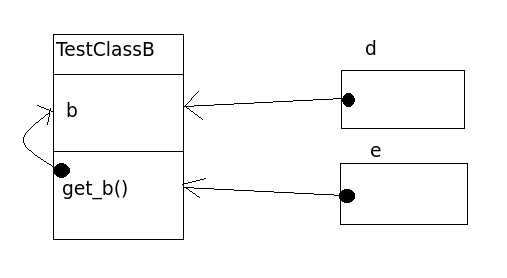# python源码学习（十二）——类中私有的变量和方法

By | 2014/08/17

python中私有变量和方法的声明方式如下：
_xxx 不能用’from module import *’导入
__xxx__ 系统定义名字
__xxx 类中的私有变量名，

python中一个变量如果声明为私有的，如下：
[code lang=”python”]
class TestClassA(object):
__a = 10
def get_a(self):
return self.__a

class TestClassB(object):
b = 10
def get_b(self):
return b
[/code]

[code lang=”python”]
a = TestClassA()
b = a.get_a()
[/code]

[code lang=”python”]
b = a.__a
[/code]

b是开辟了一块内存，内存的结构和__a完全相同，你修改b的值，但不会修改__a的值。

[code lang=”python”]
c = TestClassB()
d = c.b
e = c.get_b()
[/code]